Question

# find the area the perimeter of a rectangular wall that measures 25 feet long and 15...

find the area the perimeter of a rectangular wall that measures 25 feet long and 15 feet wide

length = 25

perimeter = 2 * (length + breadth) = 2*(25+15)=80 feet

#### Earn Coins

Coins can be redeemed for fabulous gifts.

Similar Homework Help Questions
• ### A ladder 25 feet long is leaning against the wall of a house (see figure).

Moving Ladder A ladder 25 feet long is leaning against the wall of a house (see figure). The base of the ladder is pulled away from the wall at a rate of 2 feet per second. (a) How fast is the top of the ladder moving down the wall when its base is 7 feet. 15 feet, and 24 feet from die wall?(b) Consider die triangle formed by the side of the house, the ladder, and the ground. Find the rate at...

• ### A farmer has 400 feet of fencing with which to build a rectangular pen. He will...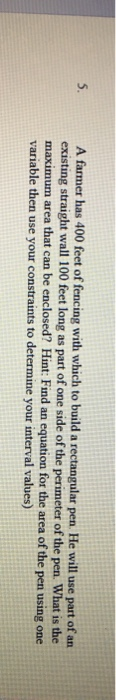A farmer has 400 feet of fencing with which to build a rectangular pen. He will use part of an existing straight wall 100 feet long as part of one side of the perimeter of the pen. What is the maximum area that can be enclosed? Hint: Find an equation for the area of the pen using one variable then use your constraints to determine your interval values)

• ### A rectangular field is three times as long as it is wide. If the perimeter of...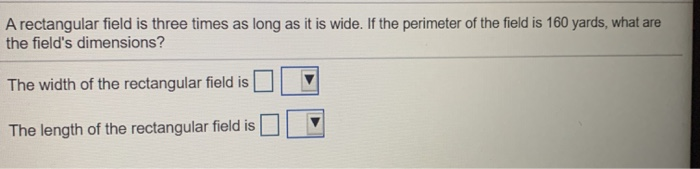A rectangular field is three times as long as it is wide. If the perimeter of the field is 160 yards, what are the field's dimensions? The width of the rectangular field is The length of the rectangular field is

• ### A large rectangular movie screen in an IMAX theater has an area of 8,500 square feet....

A large rectangular movie screen in an IMAX theater has an area of 8,500 square feet. Find the dimensions of the screen if it is 15 feet longer than it is wide. length       ft width       ft

• ### Luke has a rectangular garden which is 15 feet long and 14 feet wide. He has...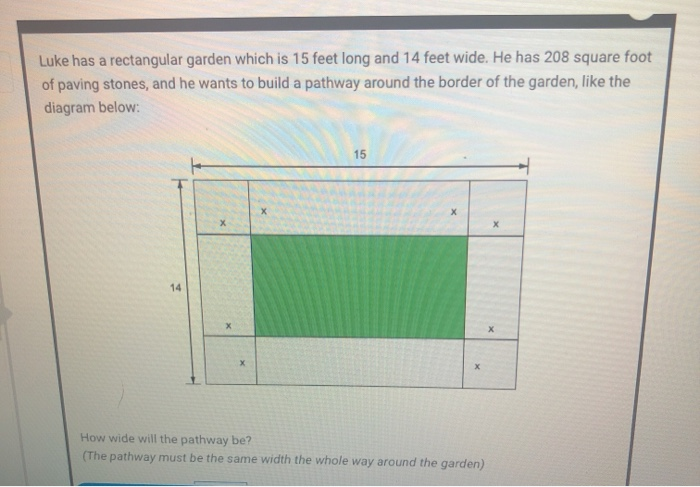Luke has a rectangular garden which is 15 feet long and 14 feet wide. He has 208 square foot of paving stones, and he wants to build a pathway around the border of the garden, like the diagram below: 15 X 14 X x How wide will the pathway be? (The pathway must be the same width the whole way around the garden)

• ### The perimeter of a rectangular window is 24 feet. The width of the window is 4...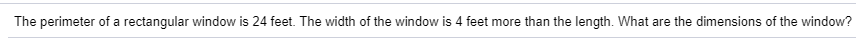The perimeter of a rectangular window is 24 feet. The width of the window is 4 feet more than the length. What are the dimensions of the window?

• ### the length of a rectangular garden is 7 feet more than three times the width. the...

the length of a rectangular garden is 7 feet more than three times the width. the perimeter of the garden is 86 feet. find the area of the garden.

• ### I need help with part C 21. Moving Ladder A ladder 25 feet long is leaning...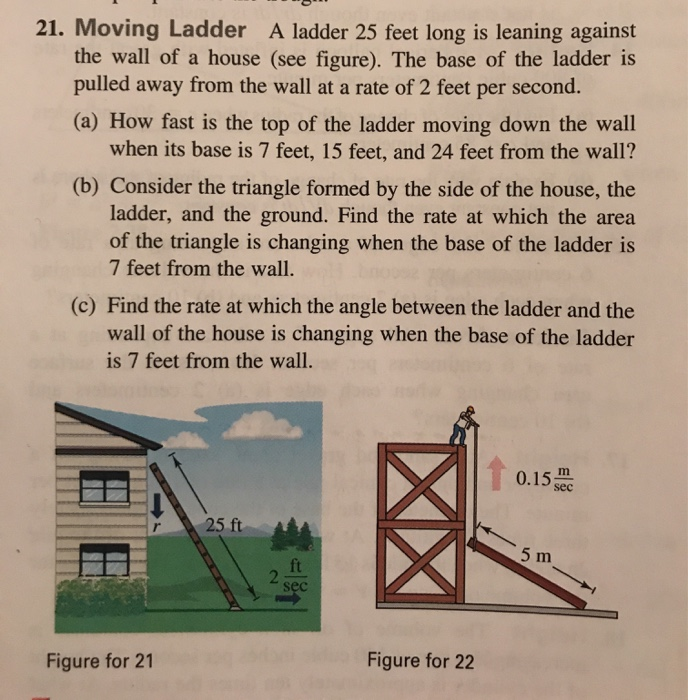I need help with part C 21. Moving Ladder A ladder 25 feet long is leaning against the wall of a house (see figure). The base of the ladder is pulled away from the wall at a rate of 2 feet per second. (a) How fast is the top of the ladder moving down the wall when its base is 7 feet, 15 feet, and 24 feet from the wall? (b) Consider the triangle formed by the side of the...

• ### Optimization Find the dimensions of a rectangle with a perimeter of 76 feet that has the...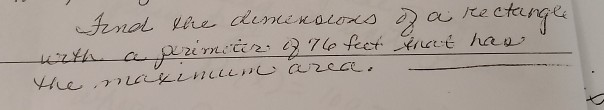Optimization Find the dimensions of a rectangle with a perimeter of 76 feet that has the maximum area. Find the dimensions of a rectange worth a primitir. 876 feet freut han the naikinillnearice.

• ### Oliver’s company needs to enclose a rectangular area of 5200 square feet on three sides with...

Oliver’s company needs to enclose a rectangular area of 5200 square feet on three sides with barbed wire fencing. Find the dimensions of that will minimize the amount of fencing required (thus minimizing building cost). Hint: minimizing the amount of fencing is equivalent to minimizing the perimeter, or distance around, the three sides of the enclosure.